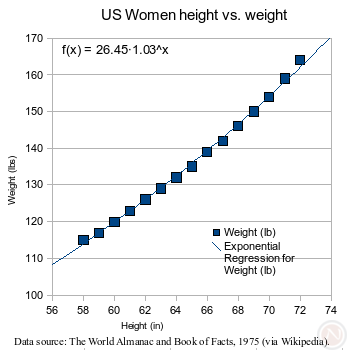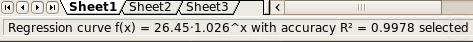Display equations for regression lines - OpenOffice.org Ninja

# Display equations for regression lines

#### Posted by Andrew Z at Saturday, December 15, 2007 | Permalink

For while, charts in Calc could do regression analysis, and starting in OpenOffice.org 2.4, there is an easy way to display the equation to the chart.In case statistics class is a fuzzy memory for you, a regression curve calculates a mathematical function to describe a set of data. Regression is helpful with predictions. For example, looking at the chart on the right. There are no values for a woman 80 inches tall, but using either the curve or its function, her height could be extrapolated to about 198 lbs.

### How to do it

1. Create an XY (Scatter) Chart.
2. Click off the chart to make sure the chart is not selected.
3. Double click the chart to enter chart edit mode.
4. Click Insert->Statistic.5. Choose the type of regression line you want.
6. Carefully right click the regression line.
7. Choose Insert Regression Curve Equation.### Increasing precision

By default, the coefficient of determination R2 and equation have little precision. To add decimal places:

1. Enter chart mode if you are not already in it.
2. Right click on the equation and/or 2
3. Select Object Properties.
4. Click the Numbers tab.
5. Choose the category Number.
6. Format as desired.

### Status bar

Since before OpenOffice.org 2.4, the regression curve equation and coefficient of determination R2 are visible by clicking on the curve.### Development snapshot

Please keep in mind I am using an early OpenOffice.org 2.4.0 development snapshot 680m239. Before the final release in March 2008, the code will be more polished.

### Compared to Microsoft ExcelThere are a few differences between Excel 2003 and OpenOffice.org.

1. The proportions of the chart change importing into Excel. The image above is from the Excel 2003 application imported from OpenOffice.org.
2. Excel uses the term trendline instead of regression curve equation.
3. Both applications allow the use of the context menu (right click) to add, clear, and configure trendlines, but OpenOffice.org also has the Insert->Statistics menu option.
4. OpenOffice.org's context menu for adding the function is limited. It simply adds a linear regression curve.
5. Excel 2003 allows changing the trendline between types (e.g., from linear to exponential) by clicking on the trendline, but in OpenOffice.org, you must choose the data series instead (issue 44768).
6. Excel 2003 shows more precision by default than OpenOffice.org, but you can add precision by right clicking on the equation. Then, choose Object Properties. Then, go the Numbers tab. Then, change Category to Number. Then, set Decimal places.
7. Excel 2003 has a few more configuration options such as moving average (issue 66819), polynomial regression (issue 20819), forecast units (issue 5085), and intercept (issue 77932). The following two images are Excel 2003.### Tidbits

This feature was originally requested in 2002 as issue 7998. With 72 votes, it is the second-highest rated enhancement or feature request scheduled for OpenOffice.org version 2.4. Issue 7998 is also fairly high on the list of all unresolved issues by number of votes.

OpenDocument 1.1 is currently the latest approved version, but the feature specification reveals the use of ODF 1.2. ODF 1.2 is scheduled for OpenOffice.org 3.0.